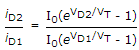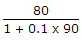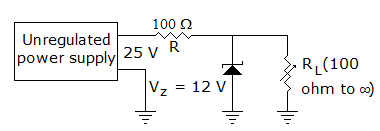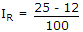# Electronics and Communication Engineering - Analog Electronics

1.

A voltage tripler circuit and voltage quadrupler circuit use identical components. Then

 A. voltage regulation in both circuits will be same B. voltage regulation in both may or may not be same C. voltage regulation in quadrupler circuit will be better than in tripler circuit D. voltage regulation in quadrupler circuit will be poorer than in tripler circuit

Explanation:

Since voltage quadrupler circuit uses more components, voltage drop will be more and voltage regulation poorer.

2.

A Ge diode operated at a junction temperature of 27°C. For a forward current of 10 mA, VD is found to be 0.3 V. If VD = 0.4 V then forward current will be

 A. 400 mA B. 475 mA C. 477 mA D. 480 mA

Explanation:iD2 = (47.73) x 10 mA = 466 mA.

3.

In a power amplifier the collector current flows for 270° of the input cycle. The operations is

 A. class A B. class B C. class AB D. class C

Explanation:

In class A operation IC exists for 360°. In class B operation IC exists for 180°. In between there is the class AB.

4.

An amplifier has input impedance of 4 kΩ and output impedance of 80 kΩ. It is used in negative feedback circuit with 10% feedback. If open loop gain is 90, the closed loop input and output impedances are

 A. 40 kΩ and 8 kΩ respectively B. 4 kΩ and 80 kΩ respectively C. 0.4 kΩ and 800 kΩ respectively D. 8 kΩ and 160 kΩ respectively

Explanation:

Closed loop input impedance = 4 (1 + 0.1 x 90) = 40 kΩ,

Closed loop Output impedance == 8 kΩ.

5.

In figure the current through resistor RA. is always equal to 130 mA B. may vary between 130 mA and 10 mA C. may vary between 65 mA and 10 mA D. is always equal to 65 mA.